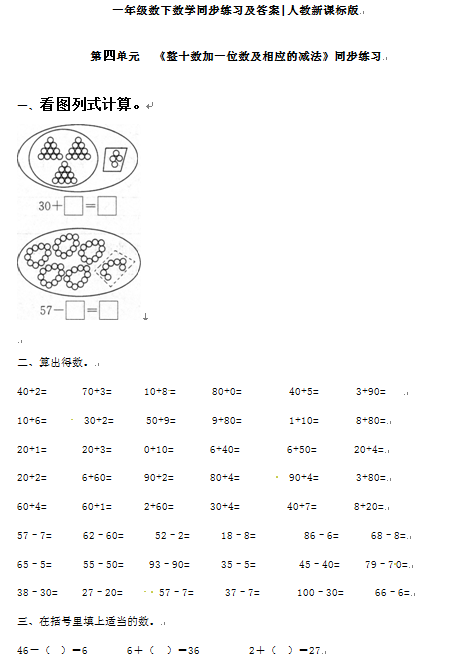40+2=       70+3=      10+8=       80+0=         40+5=       3+90=

10+6=       30+2=      50+9=       9+80=         1+10=       8+80=

20+1=       20+3=      0+10=       6+40=         6+50=       20+4=

【DOC文档3页】小学【精品】一年级下册数学同步练习-《整十数加一位数及相应的减法》1-人教新课标含答案A4电子版资料_可直接打印_会员免费下载##### 评论信息